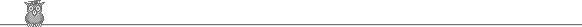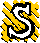### 13th Putnam 1953A1.  Show that (2/3) n3/2 < ∑1n √r < (2/3) n3/2 + (1/2) √n.A2.  The complete graph with 6 points and 15 edges has each edge colored red or blue. Show that we can find 3 points such that the 3 edges joining them are the same color.A3.  a, b, c are real, and the sum of any two is greater than the third. Show that 2(a + b + c)(a2 + b2 + c2)/3 > a3 + b3 + c3 + abc.A4.  Using sin x = 2 sin x/2 cos x/2 or otherwise, find ∫0π/2 ln sin x dx.A5.  S is a parabola with focus F and axis L. Three distinct normals to S pass through P. Show that the sum of the angles which these make with L less the angle which PF makes with L is a multiple of π.A6.  Show that √7, √(7 - √7), √(7 - √(7 + √7)), √(7 - √(7 + √(7 - √7))), ... converges and find its limit.A7.  p(x) = x3 + ax2 + bx + c has three positive real roots. Find a necessary and sufficient condition on a, b, c for the roots to be cos A, cos B, cos C for some triangle ABC.B1.  Does ∑1∞ 1/n1 + 1/n converge?B2.  p(x) is a real polynomial of degree n such that p(m) is integral for all integers m. Show that if k is a coefficient of p(x), then n! k is an integer.B3.  k is real. Solve the differential equations y' = z(y + z)k, z' = y(y + z)k subject to y(0) = 1, z(0) = 0.B4.  R is the reals. S is a surface in R3 containing the point (1, 1, 1) such that the tangent plane at any point P ∈ S cuts the axes at three points whose orthocenter is P. Find the equation of S.B5.  The coefficients of the complex polynomial z4 + az3 + bz2 + cz + d satisfy a2d = c2 ≠ 0. Show that the ratio of two of the roots equals the ratio of the other two.B6.  A and B are equidistant from O. Given k > OA, find the point P in the plane OAB such that OP = k and PA + PB is a minimum.B7.  Show that we can express any irrational number α ∈ (0, 1) uniquely in the form ∑1∞ (-1)n+1 1/(a1a2 ... an), where ai is a strictly monotonic increasing sequence of positive integers. Find a1, a2, a3 for α = 1/√2.To avoid possible copyright problems, I have changed the wording, but not the substance, of all the problems. The original text and solutions are available in: A M Gleason, R E Greenwood & L M Kelly, The William Lowell Putnam Mathematical Competition, Problems and Solutions, 1938-1964, MAA 1980. Out of print, but available in some university libraries.

Putnam home